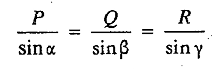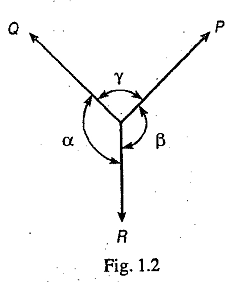# Lami's Theorem

Lami's Theorem states that if three coplaner forces acting at a point be in equilibrium, then each force is proportional to the sine of the angle between the other two forces. Mathematicallywhere P, Q and R are the three forces and α, β and γ are the angles as shown in Fig 1.2.

### You may like these posts:

1.3 co-planar concurrent forces. not co-planar forces

1.Acting at a point implies concurrent😊

2.S... Hence they r coplanar n concurrent forces

3.Can you send me all this as pdf